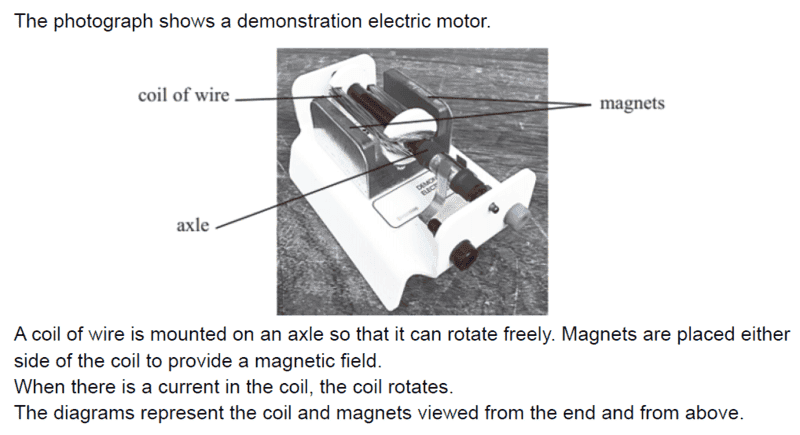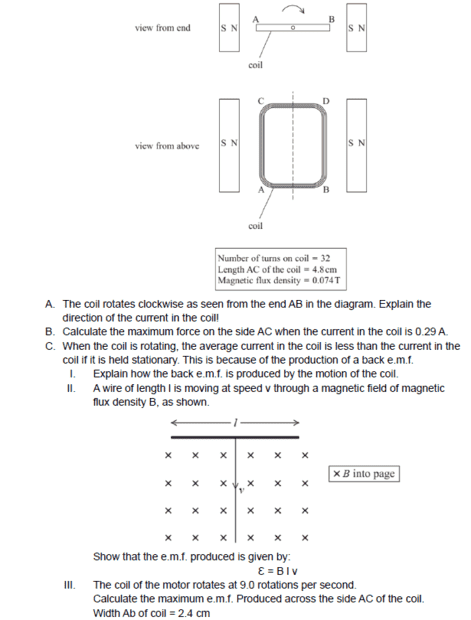# EMF produced across one side of a coil

• songoku
You calculated the emf produced by the whole coil - but you are only asked for the emf produced by side AC. Can you see why you got an answer double the correct answer?Max e.m.f produced by side AC = NBAω = 32 x 0.074 x 4.8 x 10-2 x 2.4 x 10-2 x 2π x 9 = 0.15 VIn summary, the emf produced by side AC of the coil is 0.15 V.f

#### songoku

Homework Statement
Relevant Equations
ε = - N . ΔΦ / ΔtI want to ask about part c (iii). This is what I did:

max e.m.f produced = NBAω = 32 x 0.074 x 4.8 x 10-2 x 2.4 x 10-2 x 2π x 9 = 0.15 V

But the answer is 0.077 V so my answer is off by factor of two.

Is my mistake related to "emf produced across the side of AC of the coil"?

Thanks

max e.m.f produced = NBAω = 32 x 0.074 x 4.8 x 10-2 x 2.4 x 10-2 x 2π x 9 = 0.15 V
You might like to consider the emf (including the emf's 'direction') produced by each side of the complete coil as it rotates.

•songoku
You might like to consider the emf (including the emf's 'direction') produced by each side of the complete coil as it rotates.
By direction of emf, do you mean the direction of induced current?

If yes, then the direction of induced current is anticlockwise (viewed from above) so the current on side AC is from C to A and current on side BD is from B to D

By direction of emf, do you mean the direction of induced current?
Yes. I meant the direction from positive to negative. 'Polarity' would be a better word. And this gives the current direction.

If yes, then the direction of induced current is anticlockwise (viewed from above) so the current on side AC is from C to A and current on side BD is from B to D
Agreed. Note that sides AC and BD are each producing their own emf (a bit like in part C(II) of the original question). These emf sources are in series, so the total emf is their sum.

Sides AB and CD produce no emf - do you know why?

You calculated the emf produced by the whole coil - but you are only asked for the emf produced by side AC. Can you see why you got an answer double the correct answer?

•songoku
Sides AB and CD produce no emf - do you know why?
Because point A and B are at same potential and also point C and D are at same potential?

You calculated the emf produced by the whole coil - but you are only asked for the emf produced by side AC. Can you see why you got an answer double the correct answer?
Yes, I should divide my answer by 2

Because point A and B are at same potential and also point C and D are at same potential?
These potentials are equal as you say - but that's because AB and CDproduce no emf. It is not the reason that AB and CD produce no emf.

The potentials at, say, A and C are different because an emf is induced in AC.
The potentials at, say, A and B are the same because no emf is induced in AB.

But why should emf be induced in AC but not in AB?

Yes, I should divide my answer by 2
Yes. Note that it's because AC and BD produce equal emfs and AB and CD produce no emfs.

•songoku
These potentials are equal as you say - but that's because AB and CDproduce no emf. It is not the reason that AB and CD produce no emf.

The potentials at, say, A and C are different because an emf is induced in AC.
The potentials at, say, A and B are the same because no emf is induced in AB.

But why should emf be induced in AC but not in AB?
I think because AB and CD are parallel to the magnetic field so when they rotate they don't cut the magnetic field?

By direction of emf, do you mean the direction of induced current?
An unnecessary sidenote, but I find that a strange question.
They are indeed the same here, but I always think that the emf is induced and the current IF ANY is caused by that emf. Were the coil to be incomplete - with a break in AB or CD, say - then the induced emf would be the same, but there would be no current.
When calculating the current in a motor, the current will normally be in the opposite direction to the induced emf, because that current is driven by an external emf greater than the induced back-emf.

•songoku
I think because AB and CD are parallel to the magnetic field so when they rotate they don't cut the magnetic field?
AB and CD are not always parallel to the magnetic field. But, as they rotate, they do not cut through the magnetic field in the direction required to produce an emf.

•songoku
There's a contractio on adjecto already in the title of this thead. An EMF is defined as an integral along a closed loop,
$$\mathcal{E}=\int_{\partial A} \mathrm{d} \vec{r} \cdot (\vec{E}+\vec{v} \times \vec{B})=-\dot{\Phi}_{\vec{B}},$$
where ##A## is a surface enclosed by its boundary ##\partial A##, with the velocity field ##\vec{v}## along this boundary, and
$$\Phi_{\vec{B}}=\int_A \mathrm{d}^2 \vec{f} \cdot \vec{B}$$
is the magnetic flux through this area.

•songoku
I think because AB and CD are parallel to the magnetic field so when they rotate they don't cut the magnetic field?
AB and CD are not always parallel to the magnetic field. But, as they rotate, they do not cut through the magnetic field in the direction required to produce an emf.
Another way of looking at it is this. AB and CD rotate in planes in which the magnetic field lies entirely. One might consider a motional emf across the diameter of the rod, but that would not generate a current around the closed loop.

•songoku and Steve4Physics

An EMF is defined as an integral along a closed loop,
At an introductory level it’s common to think about emfs induced in moving straight conductors. E.g. see part C-II of the original question in Post #1.

The coil’s total emf is then the sum of the emfs from the sides. So (in the context of the question) the title doesn't seem too bad.

•songoku
Thank you very much for the help and explanation Steve4Physics, Merlin3189, vanhees71, kuruman

•Steve4Physics and kuruman